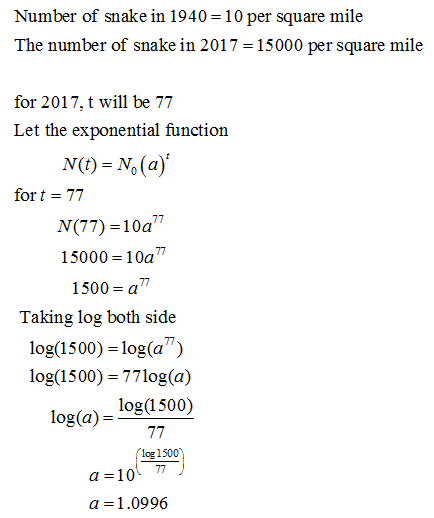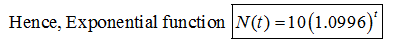# In 1940, there were about 10 brown tree snakes per square mile on the island of Guam, and in 2017, there were about 15000 per square mile.(a) Find an exponential formula for the number, ?N, of brown tree snakes per square mile on Guam ?t years after 1940?=(b) What is the growth RATE (given as a percent)?

Question
7 views

In 1940, there were about 10 brown tree snakes per square mile on the island of Guam, and in 2017, there were about 15000 per square mile.

(a) Find an exponential formula for the number, ?N, of brown tree snakes per square mile on Guam ?t years after 1940
?=

(b) What is the growth RATE (given as a percent)?

check_circle

Step 1

Given: In 1940, there were about 10 brown tree snakes per square mile on the island of Guam, and in 2017, there were about 15000 per square mile.

Step 2

(a) Find an exponential formula for the number, N, of brown tree snakes per square mile on Guam t years after 1940....

### Want to see the full answer?

See Solution

#### Want to see this answer and more?

Solutions are written by subject experts who are available 24/7. Questions are typically answered within 1 hour.*

See Solution
*Response times may vary by subject and question.
Tagged in